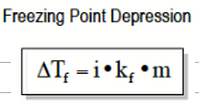# Problem: Calculate the freezing point and boiling point of a solution containing 8.10 g of ethylene glycol (C2H6O2) in 92.8 mL of ethanol. Ethanol has a density of 0.789 g/cm3.Calculate the freezing point of the solution.

###### FREE Expert Solution

The freezing point of a solution decreases with adding a solute. This is expressed in the equation below :In the equation, m = molality. So we need to calculate that before we proceed.###### Problem Details

Calculate the freezing point and boiling point of a solution containing 8.10 g of ethylene glycol (C2H6O2) in 92.8 mL of ethanol. Ethanol has a density of 0.789 g/cm3.

Calculate the freezing point of the solution.